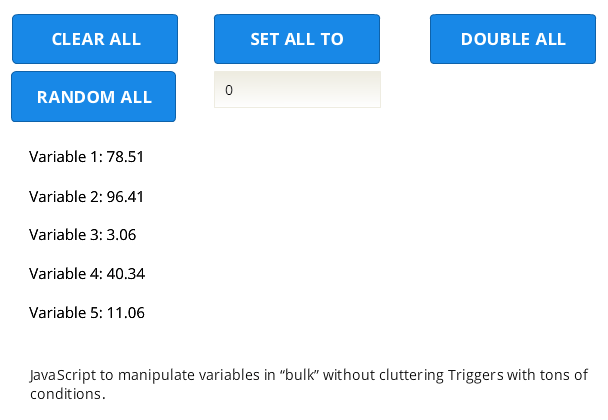# Storyline Variables and JavaScript

In Storyline 2, you have the ability to manipulate/adjust variables via triggers and conditions. You might want to increase the player’s points when collect coins or something. For each of these actions on variables, you add a trigger in Storyline to adjust the variable one by one. You don’t need JavaScript to handle variables at all.

However, there are circumstances where a simple line of JavaScript code saves you ton of work, and especially, gives you clear view of triggers in Storyline. Let’s say you have over 50 variables that you need deal with. Let’s assume these are numbers. Maybe representing 50 enemies. They could be the strength or location or what have you. You create your variables in Storyline: var1, var2, var3, var4, etc. You assign an initial value. So far, so good.

During the game/course you adjust these variables. Each time you adjust them, you add a trigger. For example, if you want all them to be 0, you would build 50 triggers to assign them to 0. If for some reason, you need to add 1 to all of them, you would build another 50 trigger to do that… It’s a lot of copy-paste and troubleshooting is not easy.

This is where a simple JavaScript comes in handy:

http://rabbitoreg.com/example/story.htmlTry the example above. In the Storyline course I have 5 variables: Var1, Var2, Var3, Var4 and Var5. But there are no triggers for adjusting variables. Each button calls a JavaScript.

For example, to set all five variables to zero:

`p=GetPlayer(); // Get the player`
```for (i=1;i<=5;i++) // Create a loop. We'll go through the loop 5 times."i" is the variable to count.
{
p.SetVar("Var"+i.toString(),0);
// In each loop, "i" changes. First, it's 1. So, the variable we set is "Var1".
// Next, "i" is 2. So, the variable we set is now "Var2"...
// toString() is just to turn the originally number "i" to a text.
}```

Now, you may say it’s actually 5 lines of code. Yes, but if you have 50 variables like Var6, Var7, Var8, etc… it would still 5 lines of code. The trick is that in JavaScript, we can create the variable name we’re adjusting in Storyline REAL TIME.

Here’s the example of randomization (between 100 and 1) of all “Var” variables.

`p=GetPlayer();`
```for (i=1;i<=5;i++)
{
p.SetVar("Var"+i.toString(),Math.random() * (100 - 1) + 1);
}```

Finally, how to double each number. For this, we need to know each Var first, then double its value.

```p=GetPlayer();
var number=p.GetVar("Number");
for (i=1;i<=5;i++)
{
p.SetVar("Var"+i.toString(),p.GetVar("Var"+i.toString())*2);
// Did you notice we can also read variables this way? GetVar will read Var1, Var2, Var3, etc.
}```

Next time I’ll show you how to use functions to be even more flexible with this approach. For example, you could have literally one line in Storyline in multiple scenes calling the randomization but each slide could set its own randomization limits like 0-100, 10-50, 40-200, etc.